# Matrixer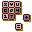econometric program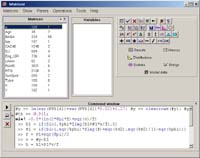System requirements Windows 95> Current version 5.1 beta

 Download Size 1.37 Mb (setup) Date August 5, 2004 How to install Matrixer Author Alexander Tsyplakov

 View help file in HTML format

 The current version of Matrixer can be used free of charge.

What's Matrixer supposed to do?

Matrixer is a piece of software especially suited for teaching econometrics and doing medium-scale applied research. It is small (in physical size, not in capabilities) and has smooth interface. Many routine operations could be done in seconds.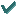The program provides many classical as well as more recent econometric techniques and models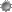Linear regressionBinomial logit and probitOrdered probitCensored (tobit) and truncated regressionPoisson and negative binomial regressionsRegression with multiplicative heteroskedasticityGARCH regressionRegression with ARMA errorsBox-Jenkins model (ARIMA) with dynamic forecastsARFIMA-FIGARCH (long-memory models)(Generalized) instrumental variables estimatorNonparametric regressionNonparametric density estimationQuantile regressionSimultaneous equations, 2SLS, 3SLS and FIMLVector autoregressionNonlinear regressionNonlinear instrumental variables methodMethod of maximum likelihoodThe program includes many other helpful features to handle and analyze dataDescriptive statistics (mean, variance, etc.)Various data plotsVisual data description (histogram, spectral density estimate, autocorrelation function)Table of correlations between variablesProbability calculator (normal, Student t, chi-square, Fisher F)Dickey-Fuller test (additional files are needed)Vector and matrix operations (that is why it was called Matrixer).Small build-in programming language for writing macrosTable editorFast drawing of function plots according to formulas3D plotsPowerful data import from text files (download examples)Data export to several popular formatsHistory of commands

What's new in Version 5.1?Major changes from Version 4.4Improved interfaceNonlinear regression with instrumental variables (generalized method of moments, GMM), command nliv!Negative binomial regression (NegBin-2), command negbin!ARFIMA-FIGARCH, command arfimafigarch!Vector autoregression, command var!Estimation of ARIMA by exact maximul likelihoodAccess to matrix parts (@-functons)Assignment result can be composite (including matrix part)Substitutions in formulas (with analitical derivatives)
Example: p := exp(z)/(1+exp(z)) >> z = @a0+@a1*xDynamic functions \$lag(), \$diff(), \$diffln(), \$csum(), \$l in formulas (with analitical derivatives)Matrices copy and paste through Windows buffer in menu of matrices"Special matrices" replaced by "model matrices"; "model scalars" and "Model data" window introducedString objects, string formulasMany functions added to work with time series: diffln(), acov(), pacf(), armaacov(), armafilter(), fdiff(), hpfilter(), genarma(), genacov(), genacovfft(), genarfima()3D plots, command plot3d!Data exportRestriction on the lengths od names of matrices, variables and macros removedHistory of commands can be editedText editor

 View modifications log file for a detailed account of changes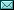E-mail: mtxr @ narod.ru